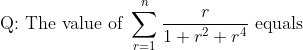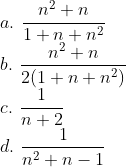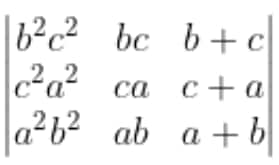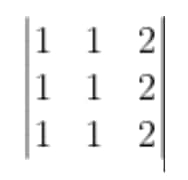# Tricks To Solve JEE Main Maths MCQs Within Seconds

###### 11 Mar'223 min read###### Synopsis

JEE Main has 20 MCQs per subject that engineering aspirants have to solve quickly to save time for other sections and score well for a good rank in the JEE Main results. Careers360 reveals a trick that can be used to solve Maths MCQs within seconds. It will help you save time and attempt more questions in your exam, and thus get a higher score.###### Synopsis

JEE Main has 20 MCQs per subject that engineering aspirants have to solve quickly to save time for other sections and score well for a good rank in the JEE Main results. Careers360 reveals a trick that can be used to solve Maths MCQs within seconds. It will help you save time and attempt more questions in your exam, and thus get a higher score.

We all are familiar with Multiple Choice Questions (MCQs). These are the questions which give us some options, usually 4, out of which one, or sometimes more than one, is correct. The Joint Entrance Examination Main, or JEE Main, has 30 Questions per subject out of which 20 are MCQs with only one correct option. Let us understand the trick with the help of some examples.

Example 1

If A + B = 45°, then find the value of (1+tanA)(1+tanB) equals

a. 1 b. 0 c. 2 d. -1

Let us first try to understand the question. It means that if A + B = 45°, then the value of (1+tanA)(1+tanB) is always constant, which is equal to one of the options. So, for all combinations of angles A and B whose sum is 45°, the value of (1+tanA)(1+tanB) will always be the same, which is equal to one of the four options.

So, whether (A = 0° and B = 45°) or (A = 1 ° and B = 44°) or (A = 10° and B = 35°), they all will give the same value of (1+tanA)(1+tanB). So, we can easily replace A and B by some convenient values of angles and get the required value of (1+tanA).(1+tanB). Let us put A = 0° and B = 45°,

(1+tanA)(1+tanB) = (1+tan0°)(1+tan45°) = (1 + 0)(1 + 1) = 1.2 = 2

So, option C is correct

Example 2Now we have to select the option that gives the correct sum for all natural number values of n. So, the correct option has to be true for n = 1, 2, 3, …and all other natural number values of n. So, if any option is giving the wrong sum for n = 1, we can be sure that that option is incorrect.

Let us try to find the options that are incorrect.

For n = 1, the actual sum of the given series is 1/(1+1+1) = 1/3

Checking what value option A for n = 1: (1+1)/(1+1+1)= 2/3. So, it gives the wrong answer for n = 1, and hence it cannot give the correct answer for all values of n. Hence this option is wrong.

Checking what value option B for n = 1: (1+1)/2(1+1+1)= 1/3. So, it gives the correct answer for n = 1. But this might give wrong answers for higher values of n. So, we will not mark this as correct right now.

Checking what value option C for n = 1: 1/(1+2)= 1/3. So, it gives the correct answer for n = 1. But this might give wrong answers for higher values of n. So, we will not mark this as correct right now.

Checking what value option D for n = 1: 1/(1+1-1)= 1. So, it gives the wrong answer for n = 1, and hence it cannot give the correct answer for all values of n. Hence this option is wrong.

Now both option A and D are eliminated, and one of option B or C is correct. Let us now see which of these two is giving the correct answer for n = 2.

For n = 2, the actual sum of the given series is

1/(1+1+1) + 2/(1+4+16) = 9/21 = 3/7

Checking what value option B for n = 2: (4+2)/2(1+2+4)= 3/7. So, it gives the correct answer for n = 2 as well. But this might give wrong answers for higher values of n. So, we will not mark this as correct right now.

Checking what value option C for n = 2: 1/(2+2)= 1/4. So, it gives the wrong answer for n = 2, and hence it cannot give the correct answer for all values of n. Hence this option is wrong.

Now we know that 3 out of 4 options are wrong, and only option B is left. So, we can safely mark option B as correct.

Example 3If the value of the determinant the given equals ka3b3c3, then the value of k is

a. 1 b. 0 c. -1 d. 2

This question means that the value of determinant always equals ka3b3c3 for all sets of values of a, b and c. In such questions, we can substitute some values of a, b and c and check to see the values of the determinant and the value of ka3b3c3. Also try to keep values of a, b and c such that ka3b3c3 does not become 0. So we will keep non-zero values of a, b and c. Let us put a = b = c = 1.

The value of determinant iswhich equals 0 (As two columns are the same) and the value of ka3b3c3 is k. So comparing these, we get k = 0.

Apart from knowing when we can apply this trick, it is also important to know when to not apply this trick. This method should not be applied to integer type or MCQ questions that have more than one correct option. Also, it should not be applied to MCQ with only one correct option, that is, ‘None of these’ or ‘All of these’.

• Joint Entrance Examination
• S
• e
• l
• p
• t
• Mathematics
• Multiple choice

## Subscribe toMembership Plan

##### *Unlock all premium content and benefits:
Read more from Latest Stories

## Careers360 helping shape your Career for a better tomorrow#### 250M+

Students#### 30,000+

Colleges#### 500+

Exams#### 1500+

E-Books